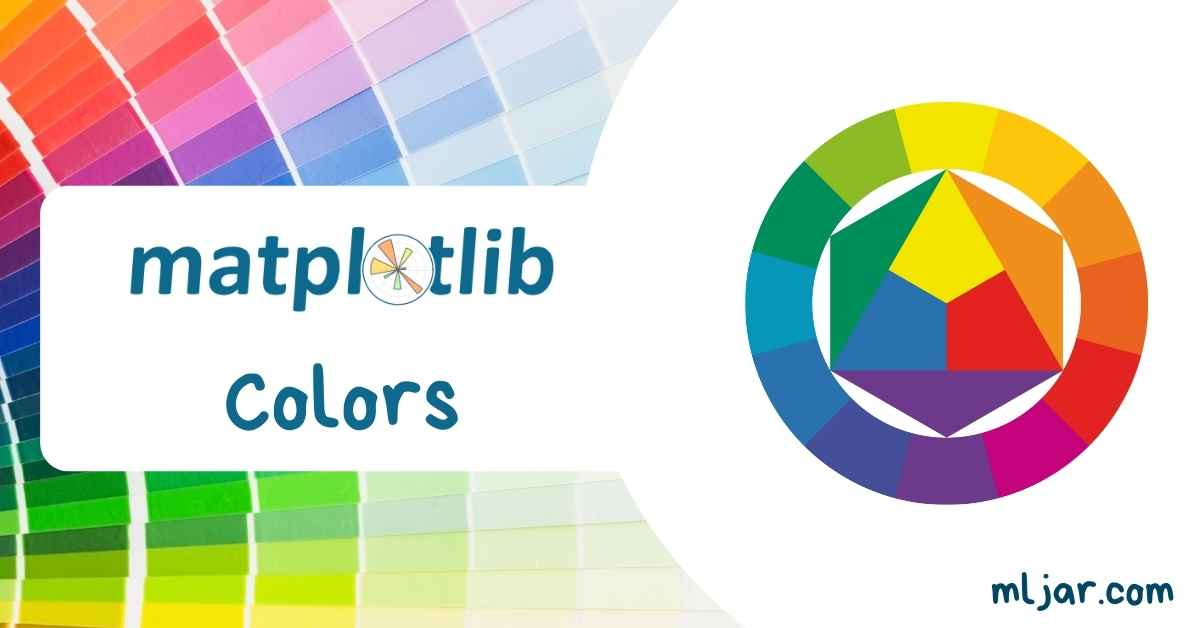`Matplotlib` is a powerful visualization package for Python. It is very customizable, thanks to this it is widly used in commercial and in academic use cases. In this article, I will show you 9 different ways how to set colors in `Matplotlib` plots. All parts of the plot can be customized with a new color. You can set colors for axes, labels, background, title. However, not every data scientist is a graphic designer that can compose nice looking colors in a single plot, so I can show you how to use predefined Matplotlib styles to get attractive plots.

## 1. Define color as RGB/RGBA float touple

The first method to define a color in a `Matplotlib` is to use RGB (red, green, blue) or RGBA (red, green, blue, alpha) touple of floats. The numbers should be in range `[0, 1]`. Each number in the touple controls how many of base color will be in final color. For example `(1, 0, 0)` is red color because there is `0` of green and blue. The alpha parameter in RGBA controls transparency. It is useful when we have overlaping elements in the plot.

Below is a minimal example of how to set color in the plot. We simply pass `color` parameter in the `plot` function with touple:

``````plt.plot(data, color=(0,1,0))
``````

Plot with several colors is presented below. RGB colors are randomly generated.

``````x = np.array(range(100))

fig = plt.figure()
ax = plt.subplot(111)

ax.spines.right.set_visible(False)
ax.spines.top.set_visible(False)

for i in range(11):
r = np.round(np.random.rand(),1)
g = np.round(np.random.rand(),1)
b = np.round(np.random.rand(),1)

y = i + np.random.rand(100)*0.25
plt.plot(x, y, color=[r,g,b])
plt.annotate(f"({r}, {g}, {b})", (100, y[-1]))
``````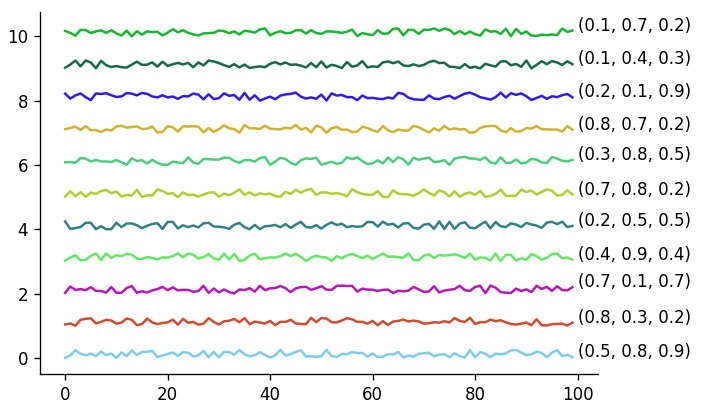Here is an example of histograms with overlaping distributions, we use alpha parameter to visualize them:

``````np.random.seed(12)
x = np.array(range(100))

fig = plt.figure()
ax = plt.subplot(111)

ax.spines.right.set_visible(False)
ax.spines.top.set_visible(False)

for i in range(4):
r = np.round(np.random.rand(),1)
g = np.round(np.random.rand(),1)
b = np.round(np.random.rand(),1)
a = np.round(np.clip(np.random.rand(), 0, 1), 1)

y = i+np.random.normal(i*3, 1.5, (4-i)*100)
plt.hist(y, color=[r,g,b, a], label=str(np.round(i*0.1, 1)))
plt.annotate(f"({r}, {g}, {b}, {a})", (i*4, (4-i)*20))
``````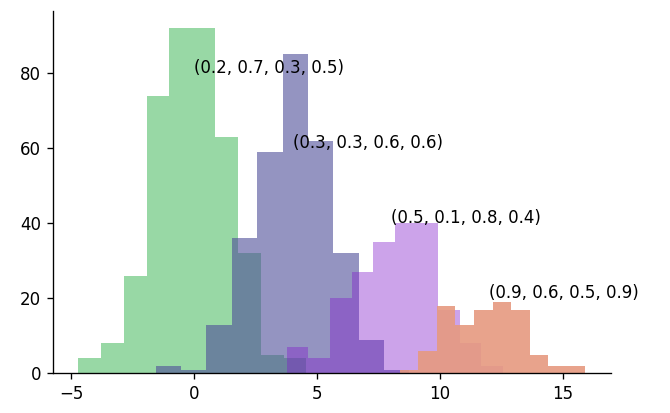## 2. Matplotlib color as RGB/RGBA hex

The RGB and RGBA colors can be defined as hex strings, similar to CSS style sheet language. The minimum value is `00` and maximum value is `FF`. You need to start string with `#`.

``````plt.plot([3,1,2,4], color="#11aa00")
plt.plot([4,2,1,3], color="#11aa0055")
plt.annotate(f"#11aa00", (0.1, 2.8))
plt.annotate(f"#11aa0055", (0.1, 3.8))
``````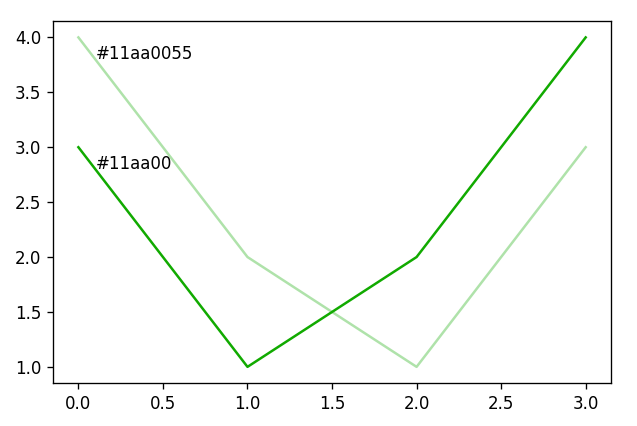## 3. Shorthand RGB/RGBA hex

The hex string can be shorted if there are the duplicate values for each channel. For example `#11aa00` can be passed as `#1a0` and `#11aa0055` can be passed as `#1a05`:

``````plt.plot([3,1,2,4], color="#1a0")
plt.plot([4,2,1,3], color="#1a05")
plt.annotate(f"#1a0", (0.1, 2.8))
plt.annotate(f"#1a05", (0.1, 3.8))
``````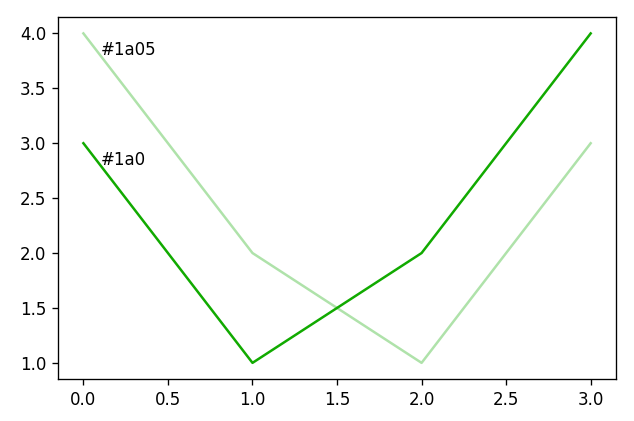Please remember, that if you are using shorthand hex, then it should be applied to all channels.

## 4. Gray scale

You can define a color as a gray scale by passing a string representation of float number from range `[0,1]`. Please remember that the float needs to be passed as a string. Below is an example of gray scale colors:

``````np.random.seed(14)
x = np.array(range(100))
fig = plt.figure()
ax = plt.subplot(111)
ax.set_facecolor('#222')
ax.spines.right.set_visible(False)
ax.spines.top.set_visible(False)

for i in range(11):
r = np.round(np.random.rand(),1)
g = np.round(np.random.rand(),1)
b = np.round(np.random.rand(),1)

y = i + np.random.rand(100)*0.25
plt.plot(x,  y, color=f"{i/10}")
plt.annotate(f"{i/10}", (100-2, y[-1]), color="w")
``````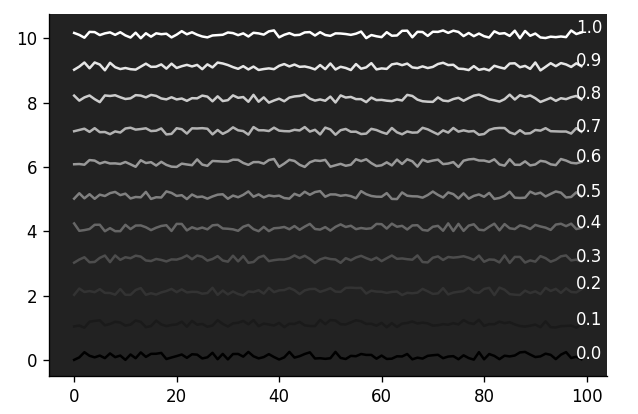## 5. Single letter string

`Matplotlib` package has implementation of several sets of named colors. The first type of named colors are single letter colors. You can list them with the following code:

``````import matplotlib.colors as mcolors
print(mcolors.BASE_COLORS)
``````

Output:

``````{'b': (0, 0, 1),
'g': (0, 0.5, 0),
'r': (1, 0, 0),
'c': (0, 0.75, 0.75),
'm': (0.75, 0, 0.75),
'y': (0.75, 0.75, 0),
'k': (0, 0, 0),
'w': (1, 1, 1)}
``````

Below is an example that is using all single letter colors:

``````colors = ['b', 'g', 'r', 'c', 'm', 'y', 'k', 'w']

np.random.seed(14)
x = np.array(range(100))
fig = plt.figure()
ax = plt.subplot(111)
ax.set_facecolor('#333')
ax.spines.right.set_visible(False)
ax.spines.top.set_visible(False)

for i in range(8):
r = np.round(np.random.rand(),1)
g = np.round(np.random.rand(),1)
b = np.round(np.random.rand(),1)

y = i + np.random.rand(100)*0.25
plt.plot(x,  y, color=colors[i])
plt.annotate(f"{colors[i]}", (100, y[-1]), color="w")

``````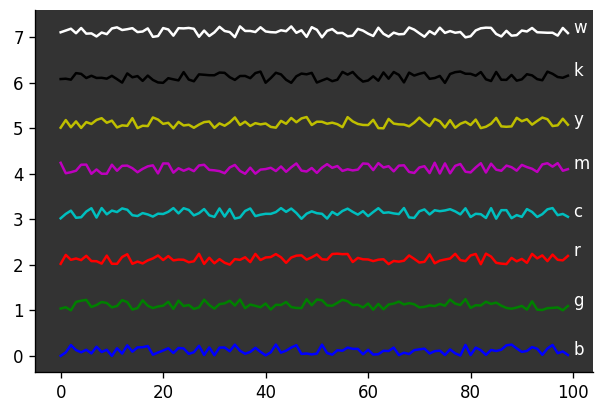## 6. X11/CSS color names

The next set of named colors comes from X11 list. Those are the same color names as used in CSS styling language (from web development). There are 148 color names available. You can list them with the following command:

``````import matplotlib.colors as mcolors
print(mcolors.CSS4_COLORS)
``````

You can check their visualization in `Matplotlib` documentation or in Wikipedia article about X11 colors.

Example color names:

``````print(mcolors.CSS4_COLORS)

>>> { 'aliceblue': '#F0F8FF',
'antiquewhite': '#FAEBD7',
'aqua': '#00FFFF',
'aquamarine': '#7FFFD4',
'azure': '#F0FFFF',
'beige': '#F5F5DC',
'bisque': '#FFE4C4',
...
``````

## 7. XKCD color names

The XKCD is a website with comics, run by Randall Munroe. He made a survey about most used RGB colors anc compiled them into the list. There are 949 colors available in the `Matplotlib` package. You can list XKCD colors with the following command:

``````import matplotlib.colors as mcolors
print(mcolors.XKCD_COLORS)

>>> { 'xkcd:cloudy blue': '#acc2d9',
'xkcd:dark pastel green': '#56ae57',
'xkcd:dust': '#b2996e',
'xkcd:electric lime': '#a8ff04',
'xkcd:fresh green': '#69d84f',
'xkcd:light eggplant': '#894585',
'xkcd:nasty green': '#70b23f',
...
``````

Please check the XKCD website to check all color names.

## 8. Cycler colors

There is colors cycler available in the `Matplotlib`. It define a list of colors that by default are used in the plot. The are used in the cycle. You can list them with following command:

``````print(plt.rcParams["axes.prop_cycle"])

>>> cycler('color', ['#1f77b4', '#ff7f0e', '#2ca02c', '#d62728', '#9467bd', '#8c564b', '#e377c2', '#7f7f7f', '#bcbd22', '#17becf'])
``````

You can observe color cycle by ploting several lines in the chart without specifying the color:

``````x = np.array(range(10))

for i in range(30):
plt.plot(x, i+x)
``````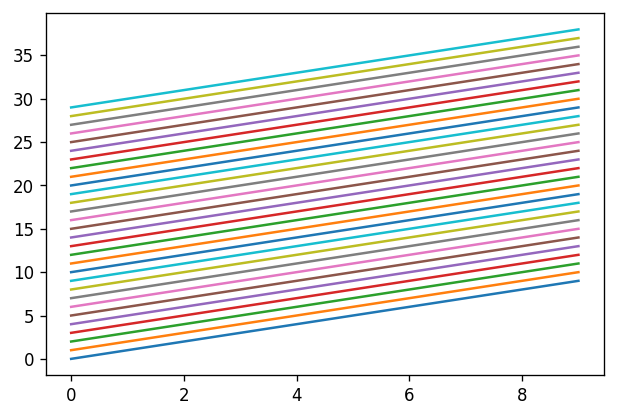You can access the cycler colors by string `"Cx"`, where `x` is a color index in the list. For example to get first color from the cycler list you will use `color="C0"` - counting starts from 0 :-)

## 9. Tableau colors

The last set of named colors is from Tableau palette. You can access them with the following code:

``````print(mcolors.TABLEAU_COLORS)

>>> { 'tab:blue': '#1f77b4',
'tab:orange': '#ff7f0e',
'tab:green': '#2ca02c',
'tab:red': '#d62728',
'tab:purple': '#9467bd',
'tab:brown': '#8c564b',
'tab:pink': '#e377c2',
'tab:gray': '#7f7f7f',
'tab:olive': '#bcbd22',
'tab:cyan': '#17becf'}
``````

## Customize colors in the Matplotlib chart

You can set a new color to any part of the `Matplotlib` chart. Let’s take a default view for very simple plot:

``````plt.plot([1,3,2,4])
``````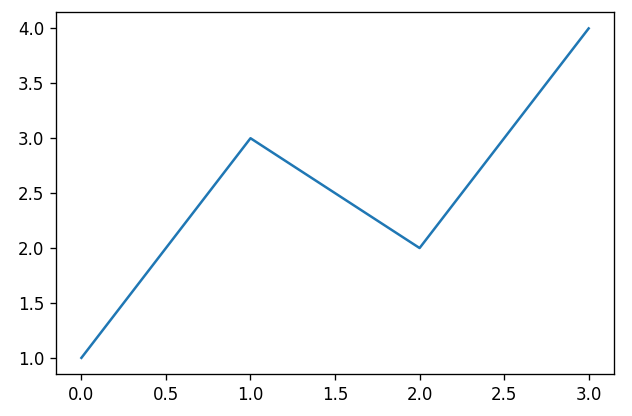We can change the background color outside the plot and inside the plot:

``````fig, ax = plt.subplots(facecolor="red")
ax.set_facecolor(color="green")
ax.plot([1,3,2,4], color="C1")
``````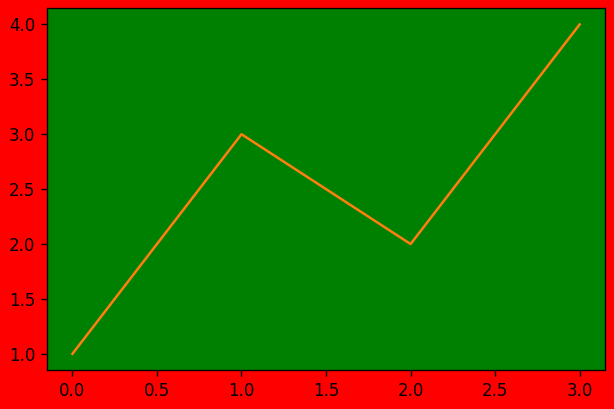We can change colors for title, labels, and ticks:

``````fig, ax = plt.subplots(facecolor="gray")
ax.set_facecolor(color="azure")
ax.set_title("Title", color="aqua")
ax.set_xlabel("X Label", color="magenta")
ax.set_ylabel('Y Label', color="orange")
ax.tick_params(labelcolor='red')
ax.plot([1,3,2,4])
``````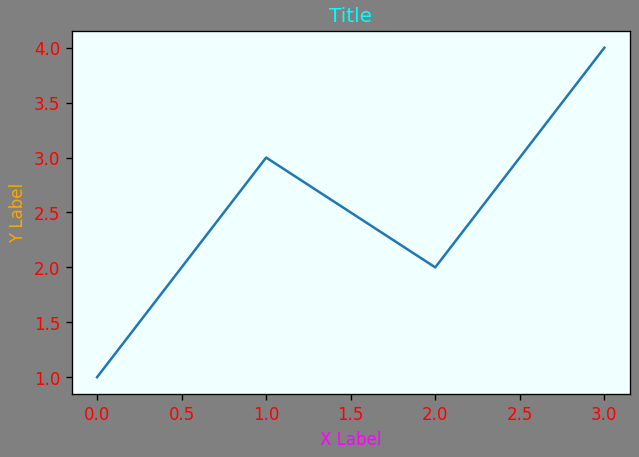## Predefined `Matplotlib` styles

There predefined styles in `Matplotlib` so you don’t need to worry which colors to select. Each style defines background color, text color and new cycles colors. It is a complete set of colors. You can list all available styles with the following code:

``````print(plt.style.available)

>>> [ 'Solarize_Light2',
'_classic_test_patch',
'_mpl-gallery',
'_mpl-gallery-nogrid',
'bmh',
'classic',
'dark_background',
'fast',
'fivethirtyeight',
'ggplot',
'grayscale',
'seaborn-v0_8',
'seaborn-v0_8-bright',
'seaborn-v0_8-colorblind',
'seaborn-v0_8-dark',
'seaborn-v0_8-dark-palette',
'seaborn-v0_8-darkgrid',
'seaborn-v0_8-deep',
'seaborn-v0_8-muted',
'seaborn-v0_8-notebook',
'seaborn-v0_8-paper',
'seaborn-v0_8-pastel',
'seaborn-v0_8-poster',
'seaborn-v0_8-talk',
'seaborn-v0_8-ticks',
'seaborn-v0_8-white',
'seaborn-v0_8-whitegrid',
'tableau-colorblind10']
``````

You can set the style globally for all plots:

``````plt.style.use("ggplot")
``````

or you can set style just for single plot:

``````with plt.style.context("ggplot"):
plt.plot([1,2,3])
``````

I’ve plotted all styles below with lines plot:

``````x = np.array(range(10))
fig = plt.figure(figsize=(10, 20), tight_layout=True)

for i, style in enumerate(['default'] + plt.style.available):
with plt.style.context(style):
ax = fig.add_subplot(10, 3, i + 1)
for i in range(10):
y = i + np.random.rand(10)*0.25
ax.plot(x,  y)

if style == "dark_background":
ax.set_title(style, color="k")
else:
ax.set_title(style)
``````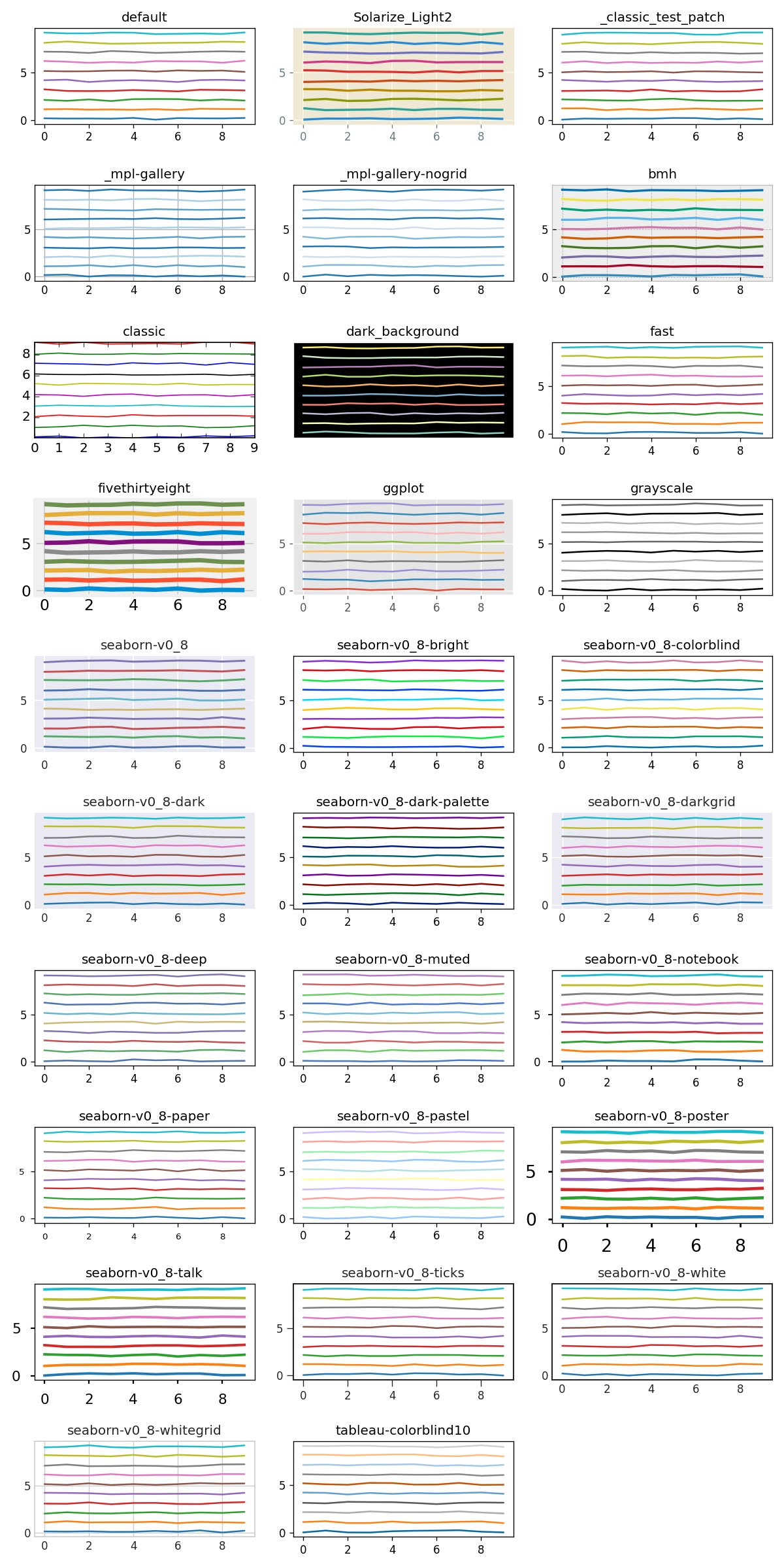All styles with histograms:

``````fig = plt.figure(figsize=(10, 20), tight_layout=True)

for i, style in enumerate(['default'] + plt.style.available):
with plt.style.context(style):
ax = fig.add_subplot(10, 3, i + 1)
for i in range(4):
y = i+np.random.normal(i*3, 1.5, (4-i)*100)
ax.hist(y)

if style == "dark_background":
ax.set_title(style, color="k")
else:
ax.set_title(style)
``````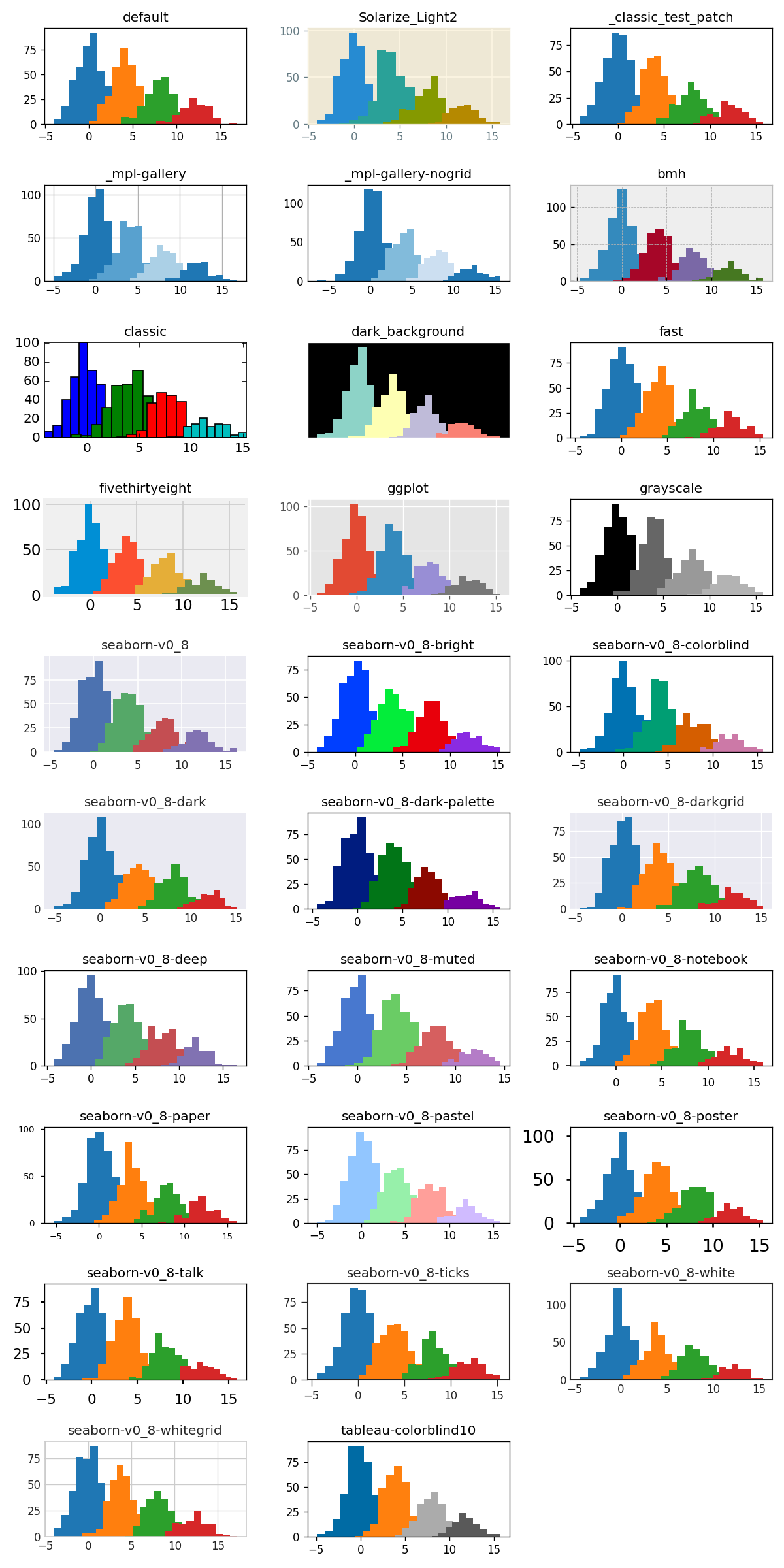## Summary

`Matplotlib` is a perfect library for scientific and commercial visualizations. It is highly customizable. There are 9 ways to set a new color. You can change color for every part of the plot. There are many predefined styles available in the package, that can help you to create attractive charts.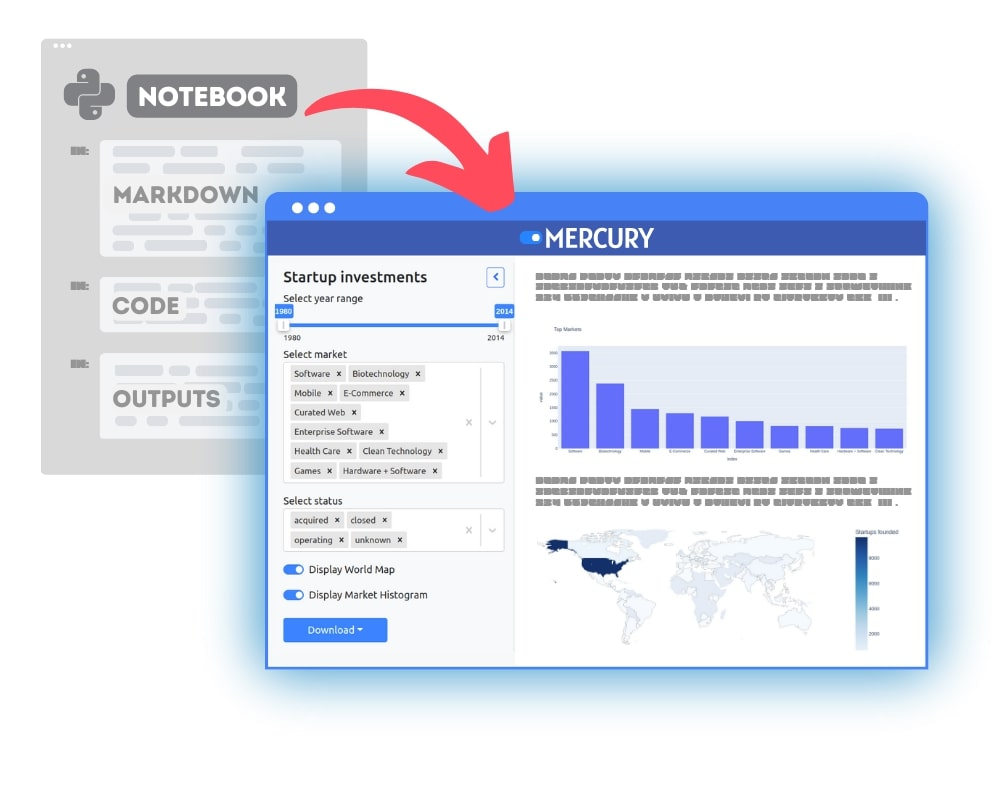### Convert Python Notebooks to Web Apps

We are working on open-source framework Mercury for converting Jupyter Notebooks to interactive Web Applications.### Articles you might find interesing